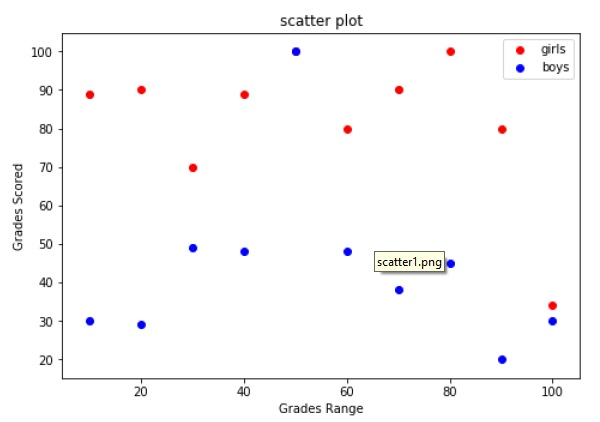# Matplotlib - Scatter Plot

Scatter plots are used to plot data points on horizontal and vertical axis in the attempt to show how much one variable is affected by another. Each row in the data table is represented by a marker the position depends on its values in the columns set on the X and Y axes. A third variable can be set to correspond to the color or size of the markers, thus adding yet another dimension to the plot.

The script below plots a scatter diagram of grades range vs grades of boys and girls in two different colors.

```import matplotlib.pyplot as plt
girls_grades = [89, 90, 70, 89, 100, 80, 90, 100, 80, 34]
boys_grades = [30, 29, 49, 48, 100, 48, 38, 45, 20, 30]
grades_range = [10, 20, 30, 40, 50, 60, 70, 80, 90, 100]
fig=plt.figure()